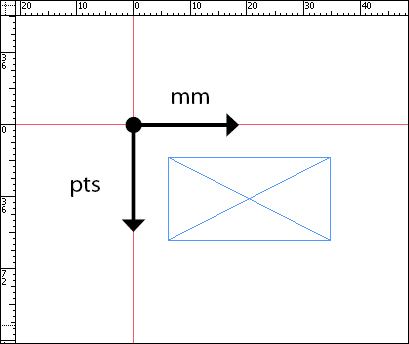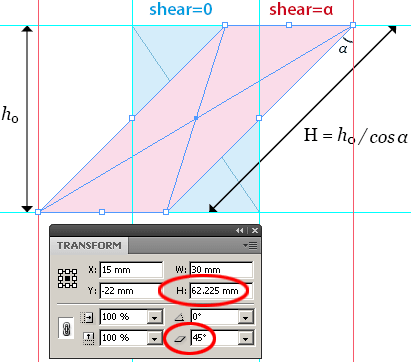## Geometric (and visible) bounds

Rather than width/height measures, most InDesign components provide a `geometricBounds` property. This information is available to every descendant of the generic `PageItem` class: `Oval`, `Rectangle`, `GraphicLine`, `Polygon`, `Group`, `Image`, `TextFrame`, etc. The `geometricBounds` property is an array. It contains the coordinates of the top left and bottom right corners of the page item's bounding box in the format [top, left, bottom, right]. (Photoshop scripters may notice the heterogeneity with respect to PS bounds format!)

Here are the four important facts to consider when you read data from `geometricBounds`:

1) They disregard the stroke of the page item. To get the coordinates of the bounding box including the stroke width, use `visibleBounds`. In the screen capture below, the yellow stroke is centered on the rectangle path. The red frame corresponds to the geometric bounds, the blue frame corresponds to the visible bounds:2) They are relative to the ruler origin (`Document.zeroPoint`), which depends on the user settings and can be changed at any moment. In the configuration below, the rectangle's `geometricBounds` will return [–54.3, –31.3, –19, 18.7]:3) They are numeric values, according to the vertical and horizontal measurement units (which also depend on the user settings and can be changed at any moment). The top/bottom values are given in the vertical units, the left/right values are given in the horizontal units. In the configuration below, the top and bottom parts of the `geometricBounds` are valued in points, while the left and right parts are valued in millimeters:4) The bounding box of a page item is the smallest rectangle that encloses the object in its current state. Consequently, the `geometricBounds` change with applied rotation angle and/or shear angle. In the screen capture below, the `geometricBounds` of the blue object match its frame, but the `geometricBounds` of the red object don't:## Getting Width/Height from the Bounds

As a primary approach, a script may infer the dimensions of a page item from its `geometricBounds` (or `visibleBounds`). Here is a naive code to do so:

```// TRACK 1 -- naive "getDims" function

function naive_getDims(/*PageItem*/obj, /*bool*/visible)
// return the [width,height] of <obj>
// according to its (geometric|visible)Bounds
{
var boundsProperty = ((visible)?'visible':'geometric')+'Bounds';
var b = obj[boundsProperty];
// width=right-left , height = bottom-top
return [ b-b , b-b ];
}

// sample code
var pItem = app.selection; // get the selected object
alert('Visible Dims: ' + naive_getDims(pItem, true));
```

In many scripting cases, `naive_getDims()` is sufficient, subject to handle untransformed page item with unambiguous measurement units. But the function will return erroneous values if the shape has rotation/shear angle(s) applied. A solution consists in temporarily cancelling the effects of the rotation/shear on the bounding box before grabbing the bounds:

```// TRACK 2 -- improved "getDims" function

function improved_getDims(/*PageItem*/obj, /*bool*/visible)
// return the [width,height] of <obj>
// according to its (geometric|visible)Bounds
{
var boundsProperty = ((visible)?'visible':'geometric')+'Bounds';

// store rotation/shear angles
var ra = obj.rotationAngle;
var sa = obj.shearAngle;

// apply 'zero' angles (temporarily)
obj.rotationAngle = 0;
obj.shearAngle = 0;

// now, get the bounds
var b = obj[boundsProperty];

// restore rotation/shear angles
obj.rotationAngle = ra;
obj.shearAngle = sa;

return [ b-b , b-b ];
}
```

`improved_getDims()` makes you sure to obtain the dimensions of the object's frame disregarding the rotation/shear effects. But. . . there is still a problem: while a rotation modifies neither the width nor the height of any frame, a shear effect increases the height by 1/cos(shearAngle) as illustrated below:So, to get the actual height of a sheared frame as displayed in the Transform panel, you need to adjust the height value extracted from the `geometricBounds`:

```// TRACK 3 -- adjusted "getDims" function

// return the [width,height] of <obj>
// according to its (geometric|visible)Bounds
{
var boundsProperty = ((visible)?'visible':'geometric')+'Bounds';

// store rotation/shear angles
var ra = obj.rotationAngle;
var sa = obj.shearAngle;

// apply 'zero' angles (temporarily)
obj.rotationAngle = 0;
obj.shearAngle = 0;

// now, get the bounds
var b = obj[boundsProperty];

// restore rotation/shear angles
obj.rotationAngle = ra;
obj.shearAngle = sa;

// calculate the width and the height
var w = b-b;
var h = (b-b) / Math.cos(sa*Math.PI/180);
return [w,h];
}
```

The expression `sa*Math.PI/180` converts the shear angle to radians (as expected by the trigonometrical functions).

## Getting Width/Height from the Inner Coordinate Space

The `adjusted_getDims()` function works like a charm, but it implies that the target is free to move. If necessary, you could embed the code in a `obj.locked` backup/restore routine to make sure that the page item isn't locked when you call the function. However, there are some circumstances where a script can't act on InDesign objects during a calculation process. For instance, a common mistake is to invoke transformations on UI objects from a modal dialog context. That won't work. In this case, `adjusted_getDims()` is unusable and you need a stronger getDims method to avoid hitting the page item.

A page item stores “the data that describes its geometry” in a specific system known as its “inner coordinate space”. Several unappreciated methods refer to coordinate spaces, as `PageItem.resolve()`, `PageItem.resize()`, `PageItem.reframe()`, `PageItem.transform()`. The first one —`resolve()`— is a powerful coordinate converter, even though its prototype looks like a puzzle.

For the moment, all we need to know is that `PageItem.resolve()` can tell us the intrinseque location of top left and right bottom corners in the inner coordinate space of the page item, disregarding transformations or rotations. We will use this syntax:

`location = myPageItem.resolve([<cornerPt>,<boxLimits>],CoordinateSpaces.innerCoordinates);`

where:

`<cornerPt>` can be an `AnchorPoint` (or an equivalent `[x,y]` array),

`<boxLimits>` is a `BoundingBoxLimits` option (`geometricPathBounds` corresponds to `geometricBounds`, `outerStrokeBounds` corresponds to `visibleBounds`).

Another important aspect is that `resolve()` returns coordinates in points whatever the `ViewPreference.horizontalMeasurementUnits` and `ViewPreference.verticalMeasurementUnits`.

Now, let's build the ultimate `getDims()` function:

```function getDims(/*PageItem*/obj, /*bool*/visibleBounds)
// Return *IN POINTS* the actual [width,height] of the page item
{
var boxLimits = BoundingBoxLimits[
(visibleBounds)?
'OUTER_STROKE_BOUNDS':
'GEOMETRIC_PATH_BOUNDS'
];

var getCoords = function(cornerPt)
{ // <this>: PageItem - return [x,y]
return this.resolve([cornerPt,boxLimits],
CoordinateSpaces.innerCoordinates);
}

// get [left,top, right,bottom] inner coordinates
var coords = getCoords.call(obj,AnchorPoint.topLeftAnchor).
concat(getCoords.call(obj,AnchorPoint.bottomRightAnchor));

// calculate the width and the height
var sa = obj.shearAngle;
var w = coords-coords;
var h = ( coords-coords ) / Math.cos(sa*Math.PI/180);
return [w,h];
}
```

Finally, if you want to convert those dimensions into specific measurement units, turn to the `UnitValue` helper class (core JavaScript).